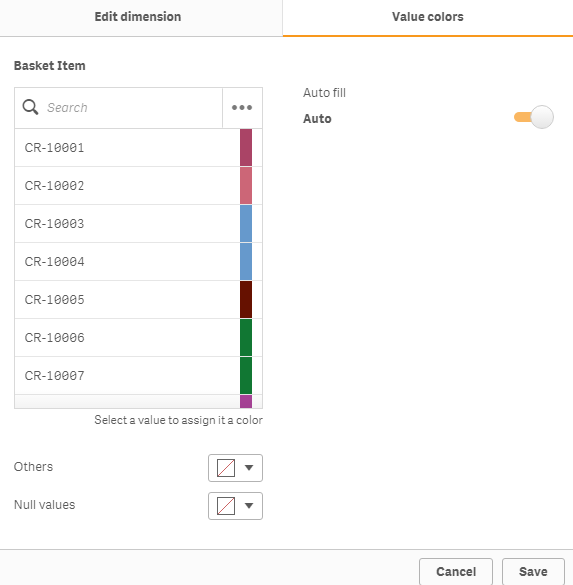# New to Qlik Sense

Discussion board where members can get started with Qlik Sense.

Contributor

## Refer Calculated Dimension Value for Color Expression Qlik Sense Pie Chart

Hi,

Can i get help  to Refer Calculated Dimension Value for Color Expression Qlik Sense Pie Chart.

=if(Status_Name='Inflow',(IF(Workflow_Age1 >= 0 and Workflow_Age1 <= 3, '0-3 Days',

IF(Workflow_Age1 >=4 and Workflow_Age1 <= 6, '4-6 Days',

IF(Workflow_Age1 >=7 and Workflow_Age1 <= 9, '7-9 Days',

IF(Workflow_Age1 > 9,  '>=10 Days' )) ) )))

I would like to have if Dimension Value = '0-3 Days' then green color... etc..,

Thanks

7 RepliesPartner

## Re: Refer Calculated Dimension Value for Color Expression Qlik Sense Pie Chart

=if( Status_Name = 'Inflow' and ( Workflow_Age1 >= 0 and Workflow_Age1 <= 3 ), green(),

and so on...

Contributor

## Re: Refer Calculated Dimension Value for Color Expression Qlik Sense Pie Chart

Use peek & match function.

Contributor

## Re: Refer Calculated Dimension Value for Color Expression Qlik Sense Pie Chart

Could you please post the formula using this

Contributor

## Re: Refer Calculated Dimension Value for Color Expression Qlik Sense Pie Chart

=IF(Workflow_Age1 >= 0 and Workflow_Age1 <= 3,

pick(match(Status_Name ,'Inflow' ),green()) and so on...

Contributor

## Re: Refer Calculated Dimension Value for Color Expression Qlik Sense Pie Chart

Color not changed using this

Contributor

## Re: Refer Calculated Dimension Value for Color Expression Qlik Sense Pie Chart

You have to use Status_Name as pie chart dimension

Highlighted
Contributor III

## Re: Refer Calculated Dimension Value for Color Expression Qlik Sense Pie Chart

in Script table:

IF(Workflow_Age1 >= 0 and Workflow_Age1 <= 3, '0-3 Days',

IF(Workflow_Age1 >=4 and Workflow_Age1 <= 6, '4-6 Days',

IF(Workflow_Age1 >=7 and Workflow_Age1 <= 9, '7-9 Days',

IF(Workflow_Age1 > 9,  '>=10 Days' )) ) ) as Workflow_Age_Days

=if(Status_Name='Inflow',Workflow_Age_Days)

Create Master Dimension with Workflow_Age_Days field and use visual color like below exp..

Drag master dimension field in your chart.